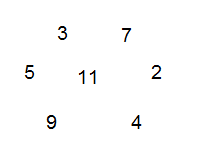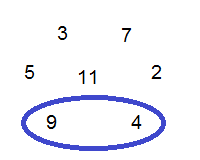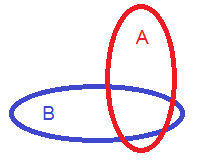## Saturday, October 3, 2015

### Conditional probabilities and Bayes' theorem

So we all know that when a sports fan asks "What chance does our team have of winning?", the speaker is asking for a probability, but when that same person later asks "What chance does our team have of winning given that John will not be playing?", the speaker is now asking for a conditional probability. In short, a conditional probability is a probability that is changed due to the addition of new information. Let's see an example.

Conditional probabilities

Let's say that we have the following set of numbers, one of which is to be picked at random with equal probability:The probability of each number being chosen is 1/7. But probabilities are usually based on subsets. So what is the probability of randomly choosing a square number from the above set?The probability is, of course, 2/7. Now comes the interesting part. Let's say that the number is still chosen at random, but you have the extra information that the number that will be chosen is going to be an even number. In other words, although you don't know which number will be chosen, you do know that it will be an even number. What is the probability that the chosen number will be a square number?Clearly the added information requires us to change the original probability of choosing a square number. We now have a smaller set of possible choices, only 2 (the red set). From these, there is only 1 square number (the intersection of the red and blue sets). So now the probability of choosing a square number is 1/2.

This is called a conditional probability. Whereas the first non-conditional probability is expressed as follows in mathematical notation:
P(number is square)
the second probability is a conditioned one and is expressed as follows:
P(number is square | number is even)
which is read as "probability that the number is square given that the number is even".

In general,
P(A|B) = P(A,B)/P(B)
where P(A|B) is the probability that event A occurs given that event B has occurred, P(A,B) is the probability that both events occur together (called the joint probability), and P(B) is the probability that event B occurred.From this, we can derive some pretty interesting equations.

Bayes' theorem

First, it is clear from the above picture that it is straightforward to define P(B|A) by simply dividing by P(A):
P(B|A) = P(A,B)/P(A)

This means that:
P(B|A) P(A) = P(A,B)
and from the other formula, that:
P(A|B) P(B) = P(A,B)
which together mean that:
P(A|B) P(B) = P(B|A) P(A)
and
P(A|B) = P(B|A) P(A)/P(B)

This last equation is known as Bayes' theorem which is something that you'll encounter all the time in probability and artificial intelligence.

In many cases, the probability P(B) is difficult to find, but we can decompose it further by noticing that the probability of selecting from set B depends on whether or not a selection was made from set A. Specifically:
P(B) = P(A) P(B|A) + P(NOT A) P(B|NOT A)
This is saying that the probability of selecting from set B is equal to the probability of one of the following events occurring:
• A selection is made from set A and it happens to also be an element in set B: P(A) P(B|A)
• A selection is not made from set A but the selected element is in set B: P(NOT A) P(B|NOT A)

Thus Bayes' theorem can be rewritten as
P(A|B) = P(A) P(B|A) / ( P(A) P(B|A) + P(NOT A) P(B|NOT A) )

This is a more practical version of the formula. Let's see a practical example of it.

Bayes' theorem in action

Let's say that you have a robot that is trying to recognise objects in front of a camera. It needs to be able to recognise you when it sees you in order to greet you and fetch you your slippers. The robot sometimes makes mistakes. It sometimes thinks that it saw you when it did not (a false positive) and it sometimes sees you and doesn't realise it (a false negative). We need to calculate how accurate it is. Let's look at the following probability tree:This tree is showing the following data:
P(you are there) = 0.1
P(you are not there) = 0.9
P(robot detects you | you are there) = 0.85
P(robot detects you | you are not there) = 0.15
P(robot does not detect you | you are there) = 0.05
P(robot does not detect you | you are not there) = 0.95


What is the probability that the robot detects you when you're there?
P(robot detects you AND you are there) =
P(robot detects you, you are there) =
P(you are there) P(robot detects you | you are there) =
0.1 x 0.85 = 0.085


Notice how we could have used the probability tree to calculate this (multiply the probabilities along a branch to AND them).

If the robot detects you, what is the probability that it is correct?
P(you are there | robot detects you) =
P(you are there) P(robot detects you | you are there) / ( P(you are there) P(robot detects you | you are there) + P(you are not there) P(robot detects you | you are not there) ) =
0.1 x 0.85 / ( 0.1 x 0.85 + 0.9 x 0.15 ) = 0.39


This is a small number, even though it correctly detects you 85% of the time. The reason is because you are in front of it only 10% of the time, which means that the majority of the time that it is trying to detect you you are not there. This will make that 15% of the time falsely detecting you pile up. One way to increase the accuracy is to limit the number of times an attempted detection is made in such a way that the probability that you are actually there is increased.

Bayesian inference

There is more to Bayes' theorem than using it to measure the accuracy of a robot's vision. It has interesting philosophical implications in epistemology. This is because it can be used to model the acquisition of knowledge. When used in this way we say that we are performing Bayesian inference. Let's say that you're a detective collecting clues on who committed a murder. You have a suspect in mind that you believe is the murderer with a certain probability. You find a clue which you believe is evidence that incriminates the suspect. This evidence should now increase your probability that the suspect is the murderer. But how do you find the new probability? Enter Bayes' theorem.

The probability you assigned to the suspect before the new evidence is P(H), the probability of the hypothesis, also known as the prior probability.
The new probability that you should assign to the suspect after discovering the evidence is P(H|E), also known as the posterior probability.
Now we use Bayesian inference to calculate the posterior probability as follows:

P(H|E) = P(H)P(E | H) / ( P(H)P(E | H) + P(NOT H)P(E | NOT H) )

The interpretation of this makes sense. The new probability given the evidence depends on two things:
• The likelihood that the suspect was the murderer. The smaller this is, the stronger the evidence needs to be to make the hypothesis likely. This is described exactly by the quote "Extraordinary claims require extraordinary evidence".
• The probability that the evidence would exist given that the suspect was not the murderer. It could be that the evidence actually supports the null-hypothesis, that is, that the suspect is actually not the murderer. This is determined by comparing the probability of the hypothesis with the probability of the null-hypothesis.

Finally notice also that if you have multiple hypothesis and want to see which is the most likely given a new evidence, we are essentially trying to find the maximum posterior probability of each hypothesis given the same evidence. Given the multiple competing hypothesis H_1, H_2, H_3, etc., the most likely H_i is found by:
argmax_i ( P(H_i)P(E | H_i) / ( P(H_i)P(E | H_i) + P(NOT H_i)P(E | NOT H_i) ) )
But we can simplify this by remembering that the denominator is P(E):
argmax_i ( P(H_i)P(E | H_i) / P(E) )
And of course since P(E) is a constant for each hypothesis, it will not affect which hypothesis will give the maximum posterior probability, so we can leave it out, giving:

argmax_i P(H_i)P(E | H_i)